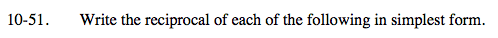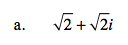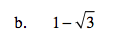### Home > A2C > Chapter Ch10 > Lesson 10.1.2 > Problem10-51

10-51.$\frac{1}{\sqrt{2}+\sqrt{2}\textit{i}}\cdot \frac{\sqrt{2}-\sqrt{2}\textit{i}}{\sqrt{2}-\sqrt{2}\textit{i}}$

$\frac{\sqrt{2}-\sqrt{2}\textit{i}}{4}=\frac{\sqrt{2}}{4}-\frac{\sqrt{2}}{4}\textit{i}$See part (a).

$\frac{-1-\sqrt{3}}{2}=-\frac{1}{2}-\frac{\sqrt{3}}{2}$